### Home > CALC > Chapter Ch2 > Lesson 2.3.2 > Problem2-118

2-118.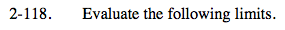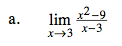Factor the numerator.

Simplify.

A limit exists at a hole. What is the y-value of the hole?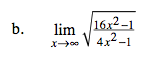Notice that this is a limit x→∞. Compare the greatest powers of the numerator and denominator.

$\lim_{x\rightarrow \infty }\sqrt{\frac{16x^{2}}{4x^{2}}}=\lim_{x\rightarrow \infty }\frac{4x}{2x}=$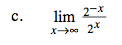$\text{Recall that } 2^{-x}=\frac{1}{2^x}$

$\lim_{x\rightarrow \infty }\frac{1}{2^{2x}}=\lim_{x\rightarrow \infty }\frac{1}{4^x}=$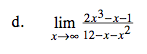This is another limit x→∞. Compare the terms with the highest powers in the numerator and denominator.

$\lim_{x\rightarrow \infty }\frac{2x^3}{-x^{2}}=\lim_{x\rightarrow \infty }\frac{2x}{-1}=$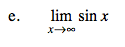Think about the graph of y = sinx. It oscillates as x→∞. This means that it does not approaches a finite value AND does not approach ±∞. When a function oscillates, we say the limit does not exist.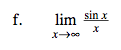Consider the numerator and the denominator separately.

As x→∞, y = sinx oscillates between y = −1 and y = 1.
As x→∞, y = x approaches ∞.
So this ratio has small values on the top and infinity on the bottom.